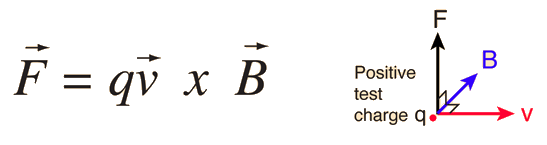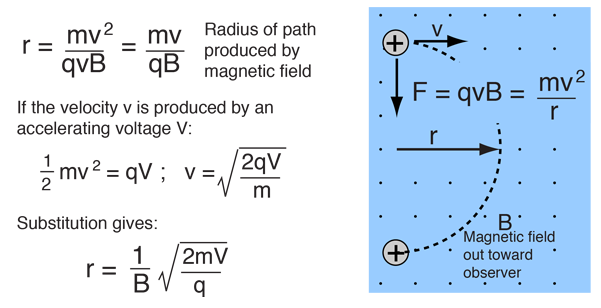# Magnetic Confinement

Since the magnetic force is always perpendicular to the velocitya charged particle will be bent into a circular path by the component of magnetic field which is perpendicular to the velocity. Any motion parallel to the magnetic field will be unchanged by it, so the general motion would be a helical motion. If B is perpencicular to v then qvB is the centripetal force and the radius of the resulting circular path is given byThis confines the charge in a orbit in a localized region of space so that it doesn't interact with container walls. This is very useful for the hot plasmas used in nuclear fusion.

 Magnetic interactions with charge Magnetic force applications
Index

Electromagnetic force

Magnetic field concepts

 HyperPhysics***** Electricity and Magnetism R Nave
Go Back